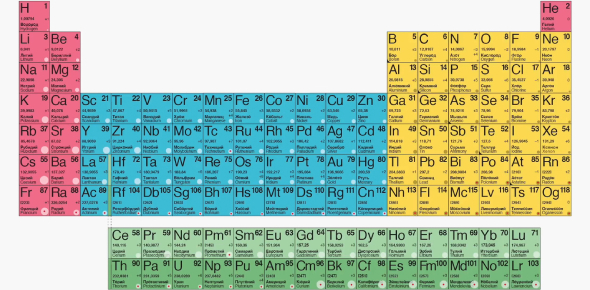# Atomic Theory & Periodic Table Assessment Quiz

30 Questions | Attempts: 57
ShareSettingsHow well do you know the Atomic Theory and Periodic Table? You can take this informative assessment quiz to test your knowledge. If you are a chemistry pro, and atoms, elements, and their properties are on your tips, you'll have a fun time taking this quiz. Test your knowledge and challenge yourself on this quiz. Just go for it, and ace it! All the best! Do not forget to share the quiz with your science lovers' friends and others.

• 1.
Protons are found in the ________.
• A.

Nucleus

• B.

Electron Cloud

• C.

Atom

• D.

Electron Energy Levels

• 2.
Neutrons are found in the ________.
• A.

Nucleus

• B.

Electron Cloud

• C.

Atom

• D.

Electron Energy Levels

• 3.
Electrons are found in the ________.
• A.

Nucleus

• B.

Electron Cloud

• C.

Atom

• D.

None of the above

• 4.
Valence electrons are found in the _____.
• A.

Inner Levels of Electrons

• B.

Outer Level of Electrons

• C.

Nucleus

• D.

Middle Levels of Electrons

• 5.
You can identify the number of valence electrons in an atom of an element from the ______
• A.

Atomic Number

• B.

Period Number

• C.

Group Number

• D.

Atomic Mass Number

• 6.
You can identify the number of energy levels of electrons in an atom from the ______
• A.

Atomic Number

• B.

Period Number

• C.

Group Number

• D.

Atomic Mass Number

• 7.
You can determine the number of  neutrons in an atom by using which formula listed below?
• A.

Atomic Number - Rounded Atomic Mass

• B.

Atomic Number - Atomic Number

• C.

Rounded Atomic Mass/Atomic Number

• D.

Rounded Atomic Mass - Atomic Number

• 8.
The charge of a electron is _______
• A.

Negative

• B.

Positive

• C.

Neutral

• D.

None of the above

• 9.
The charge of a neutron is _______
• A.

Negative

• B.

Positive

• C.

Neutral

• D.

None of the above

• 10.
Group 18 elements are known as the ________.
• A.

Alkali Metals

• B.

Alkaline Earth Metals

• C.

Noble Gases

• D.

Mixed Groups

• E.

Halogens

• F.

Noble Gases

• 11.
Group 1 elements are known as the ________.
• A.

Alkali Metals

• B.

Alkaline Earth Metals

• C.

Transition Metals

• D.

Mixed Groups

• E.

Halogens

• F.

Noble Gases

• 12.
Group 3-12 is also known as the ________.
• A.

Alkali Metals

• B.

Alkaline Earth Metals

• C.

Transition Metals

• D.

Mixed Groups

• E.

Halogens

• F.

Noble Gases

• 13.
Group 17 elements are known as the ________.
• A.

Alkali Metals

• B.

Alkaline Earth Metals

• C.

Transition Metals

• D.

Mixed Groups

• E.

Halogens

• F.

Noble Gases

• 14.
Group 13-16 is also known as the ________.
• A.

Alkali Metals

• B.

Alkaline Earth Metals

• C.

Transition Metals

• D.

Mixed Groups

• E.

Halogens

• F.

Noble Gases

• 15.
Calcium has an atomic mass of 40 and an atomic number of 20. How many neutrons are there in one atom of calcium?
• A.

50

• B.

35

• C.

20

• D.

25

• 16.
Which group contains elements found in all three states of matter?
• A.

Boron Group

• B.

Noble Gases

• C.

Transition Metals

• D.

Halogens

• 17.
The atomic number of an element tells you the number of ____.
• A.

Electrons

• B.

Neutrons & Electrons

• C.

Protons, Electrons & Neutrons

• D.

Protons & Electrons

• 18.
How many electrons are in the outer most orbital of Selenium?
• A.

8

• B.

3

• C.

7

• D.

6

• 19.
If an element can be drawn into thin wire, it is called ____.
• A.

Weak

• B.

Malleable

• C.

Flexible

• D.

Ductile

• 20.
Vertical Columns on the periodic table are called ____.
• A.

Rows

• B.

Period

• C.

Groups

• D.

Elements

• 21.
The scientist who discovered that electrons of an atom travel in very specific paths or orbitals around the nucleus.
• A.

J.J. Thomson

• B.

Ernest Rutherford

• C.

Niels Bohr

• D.

John Dalton

• 22.
Most of the elements on the periodic table are ____.
• A.

Metalloids

• B.

Noble Gases

• C.

Metals

• D.

Nonmetals

• 23.
Which of the following is a nonmetal and a liquid?
• A.

Mercury

• B.

Bromine

• C.

Gallium

• D.

Hydrogen

• 24.
Which of the following is NOT a solid?
• A.

Calcium

• B.

Phosphorus

• C.

Nitrogen

• D.

Potassium

• 25.
How many elements are in Period 3?
• A.

2

• B.

8

• C.

18

• D.

11

## Related TopicsBack to top
×

Wait!
Here's an interesting quiz for you.